# Problem 55825. Measure the hydraulic conductivity with a constant-head permeameter

A constant-head permeameter is a device for measuring the hydraulic conductivity K of a soil sample. In this problem the sample is placed in a cylinder of length L and cross-sectional area A. By supplying a flow Q to a standpipe, water is maintained at a constant level so that the head difference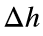, or the difference in water levels between the standpipe and outlet, is constant.
The hydraulic conductivity can then be determined from Darcy’s lawwhere the head gradient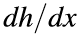is simply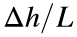. Darcy’s law applies when a Reynolds number based on the specific discharge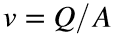and representative diameter d of the soil grains is less than (approximately) 1—that is,where ν is the kinematic viscosity of the fluid.
The Kozeny-Carman equation provides one way to relate the hydraulic conductivity to the representative grain diameter:where g is the acceleration of gravity and n is the porosity of the soil
Write a function that takes as input the flowrate, the head difference, porosity, and length and diameter of the cylinder holding the soil sample and returns the hydraulic conductivity and a flag indicating whether Darcy’s law is valid. Compute the conductivity using Darcy’s law regardless of its validity, and use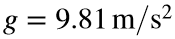and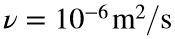.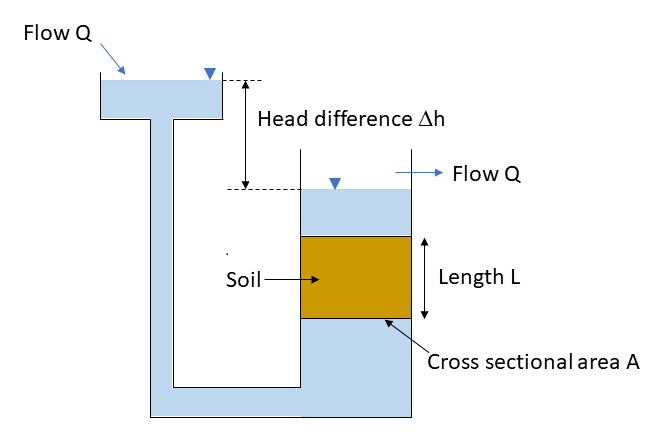### Solution Stats

33.33% Correct | 66.67% Incorrect
Last Solution submitted on Aug 31, 2023

### Community Treasure Hunt

Find the treasures in MATLAB Central and discover how the community can help you!

Start Hunting!